# Mean Reversion in Time SeriesTime series data is simply a collection of observations generated over time. For example, the speed of a race car at each second, daily temperature, weekly sales figures, stock returns per minute, etc. In the financial markets, a time series tracks the movement of specific data points, such as a security’s price over a specified period of time, with data points recorded at regular intervals. A time series can be generated for any variable that is changing over time. Time series analysis comprises of techniques for analyzing time series data in an attempt to extract useful statistics and identify characteristics of the data. Time series forecasting is the use of a mathematical model to predict future values based on previously observed values in the time series data.

The graph shown below represents the daily closing price of Aluminium futures over a period of 93 trading days, which is a time series.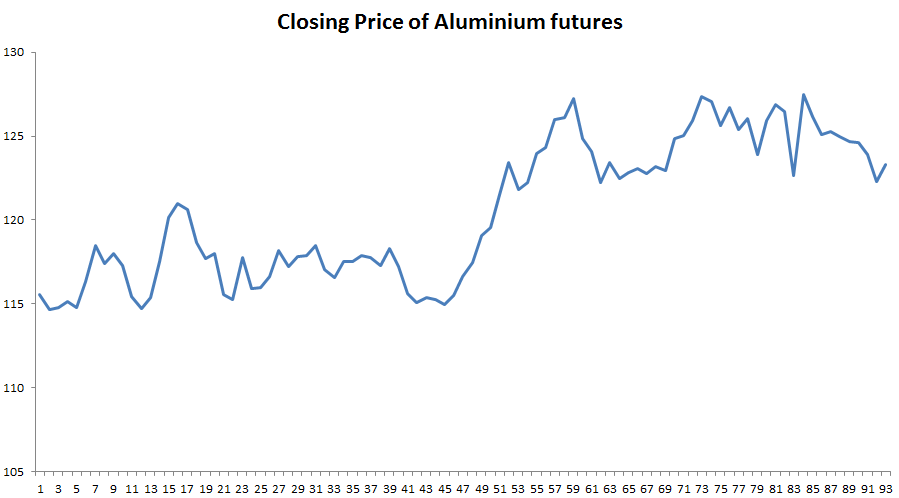### Mean Reversion

Mean reversion trading is the theory which suggests that prices, returns, or various economic indicators tend to move to the historical average or mean over time. This theory has led to many trading strategies which involve the purchase or sale of a financial instrument whose recent performance has greatly differed from their historical average without any apparent reason. For example, let the price of gold increase on average by INR 10 every day and one day the price of gold increases by INR 40 without any significant news or factor behind this rise, then by the mean reversion principle we can expect the price of gold to fall in the coming days such that the average change in price of gold remains the same. In such a case, the mean reversionist would sell gold, speculating the price to fall in the coming days. Thus, making profits by buying the same amount of gold he had sold earlier, now at a lower price.

A mean-reverting time series has been plotted below, the horizontal black line represents the mean and the blue curve is the time series which tends to revert back to the mean.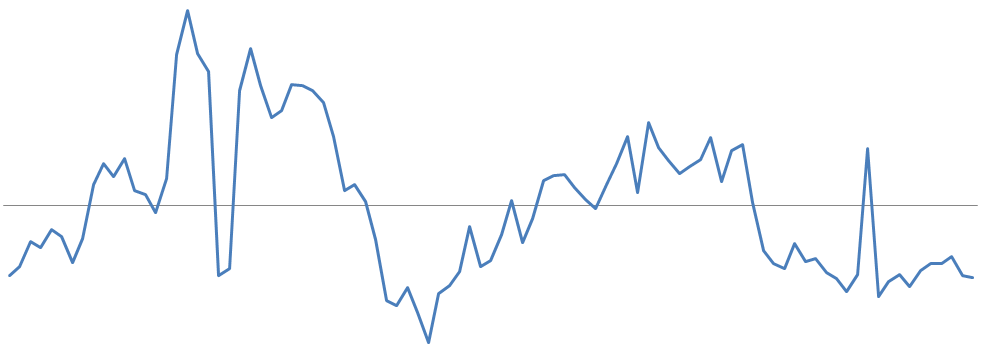A collection of random variables is defined to be a stochastic or random process. A stochastic process is said to be stationary if its mean and variance are time invariant (constant over time). A stationary time series will be mean reverting in nature, i.e. it will tend to return to its mean and fluctuations around the mean will have roughly equal amplitudes. A stationary time series will not drift too far away from its mean because of its finite constant variance. A non-stationary time series, on the contrary, will have a time varying variance or a time varying mean or both, and will not tend to revert back to its mean. In the financial industry, traders take advantage of stationary time series by placing orders when the price of a security deviates considerably from its historical mean, speculating the price to revert back to its mean. They start by testing for stationarity in a time series. Financial data points, such as prices, are often non-stationary, i.e. they have means and variances that change over time. Non-stationary data tends to be unpredictable and cannot be modeled or forecasted. A non-stationary time series can be converted into a stationary time series by either differencing or detrending the data. A random walk (the movements of an object or changes in a variable that follow no discernible pattern or trend) can be transformed into a stationary series by differencing (computing the difference between Yt and Yt -1). The disadvantage of this process is that it results in losing one observation each time the difference is computed. A non-stationary time series with a deterministic trend can be converted into a stationary time series by detrending (removing the trend). Detrending does not result in loss of observations. A linear combination of two non-stationary time series can also result in a stationary, mean-reverting time series. The time series (integrated of at least order 1), which can be linearly combined to result in a stationary time series are said to be cointegrated.

Shown below is a plot of a non-stationary time series with a deterministic trend (Yt = α + βt + εt) represented by the blue curve and its detrended stationary time series (Yt - βt = α + εt) represented by the red curve.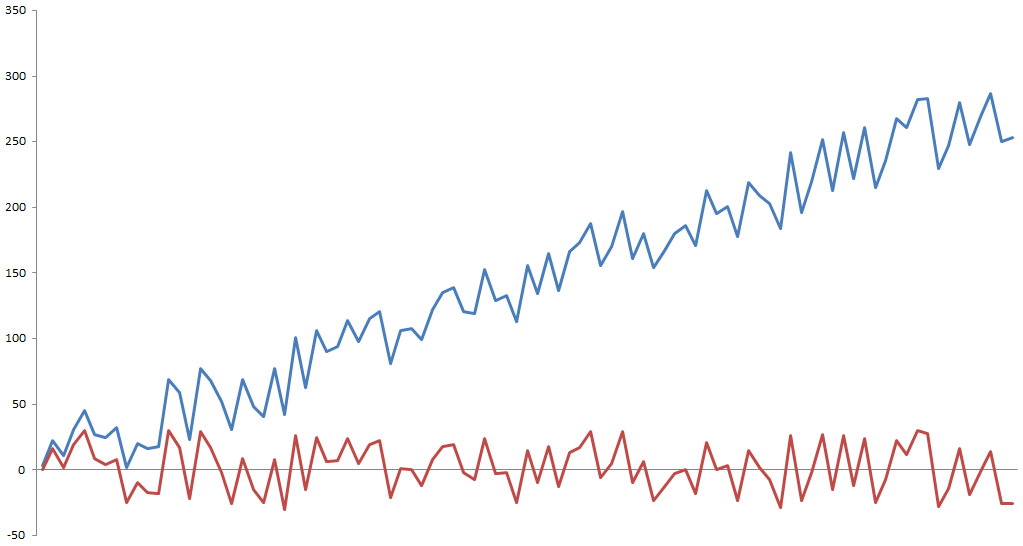### Trading Strategies based on Mean Reversion

One of the simplest mean reversion trading related trading strategies is to find the average price over a specified period, followed by determining a high-low range around the average value from where the price tends to revert back to the mean. The trading signals will be generated when these ranges are crossed - placing a sell order when the range is crossed on the upper side and a buy order when the range is crossed on the lower side. The trader takes contrarian positions, i.e. goes against the movement of prices (or trend), expecting the price to revert back to the mean. This strategy looks too good to be true and it is, it faces severe obstacles. The lookback period of the moving average might contain a few abnormal prices which are not characteristic to the dataset, this will cause the moving average to misrepresent the security’s trend or the reversal of a trend. Secondly, it might be evident that the security is overpriced as per the trader’s statistical analysis, yet he cannot be sure that other traders have made the exact same analysis. Because other traders don’t see the security to be overpriced, they would continue buying the security which would push the prices even higher. This strategy would result in losses if such a situation arises.

Pairs Trading is another strategy that relies on the principle of mean reversion trading. Two co-integrated securities are identified, the spread between the price of these securities would be stationary and hence mean reverting in nature. An extended version of Pairs Trading is called Statistical Arbitrage, where many co-integrated pairs are identified and split into buy and sell baskets based on the spreads of each pair. The first step in a Pairs Trading or Stat Arb model is to identify a pair of co-integrated securities. One of the commonly used tests for checking co-integration between a pair of securities is the Augmented Dickey-Fuller Test (ADF Test). It tests the null hypothesis of a unit root being present in a time series sample. A time series which has a unit root, i.e. 1 is a root of the series’ characteristic equation, is non-stationary. The augmented Dickey-Fuller statistic, also known as t-statistic, is a negative number. The more negative it is, the stronger the rejection of the null hypothesis that there is a unit root at some level of confidence, which would imply that the time series is stationary. The t-statistic is compared with a critical value parameter, if the t-statistic is less than the critical value parameter then the test is positive and the null hypothesis is rejected.

### Co-integration check - ADF Test

Consider the Python code shown below for checking co-integration: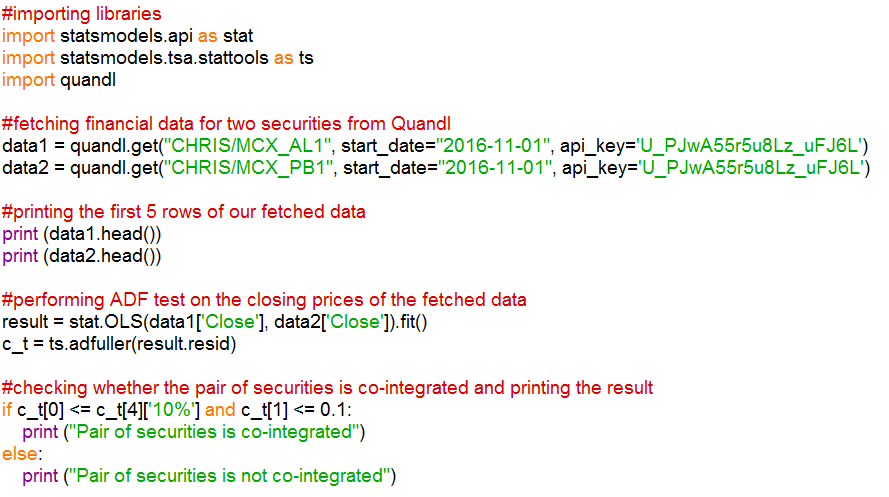We start by importing relevant libraries, followed by fetching financial data for two securities using the quandl.get() function. Quandl provides financial and economic data directly in Python by importing the Quandl library. In this example, we have fetched data for Aluminium and Lead futures from MCX. We then print the first five rows of the fetched data using the head() function, in order to view the data being pulled by the code. Using the statsmodels.api library, we compute the Ordinary Least Squares regression on the closing price of the commodity pair and store the result of the regression in the variable named ‘result’. Next, using the statsmodels.tsa.stattools library, we run the adfuller test by passing the residual of the regression as the input and store the result of this computation the array “c_t”. This array contains values like the t-statistic, p-value, and critical value parameters. Here, we consider a significance level of 0.1 (90% confidence level). “c_t” carries the t-statistic, “c_t” contains the p-value and “c_t” stores a dictionary containing critical value parameters for different confidence levels. For co-integration we consider two conditions, firstly we check whether the t-stat is lesser than the critical value parameter (c_t <= c_t['10%']) and secondly whether the p-value is lesser than the significance level (c_t <= 0.1). If both these conditions are true, we print that the “Pair of securities is co-integrated”, else print that the “Pair of securities is not cointegrated”.

### Output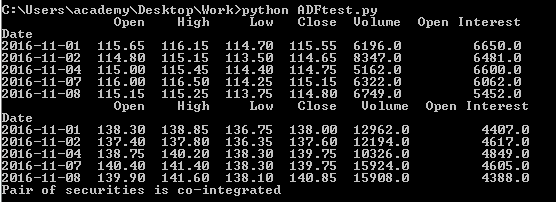To know more about Pairs Trading, Statistical Arbitrage and the ADF test you can check out the self-paced online certification course on “Statistical Arbitrage Trading“ offered jointly by QuantInsti and MCX to learn how to trade Statistical Arbitrage strategies using Python and Excel.